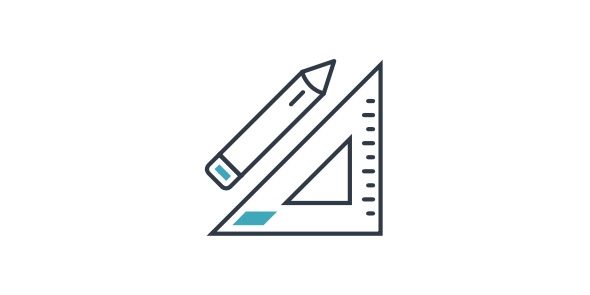15 Questions | Total Attempts: 134Settings• 1.
Which pair of numbers has a product of 50 and a quotient of 2?
• A.

25, 2

• B.

12.5, 4

• C.

1, 50

• D.

10, 5

• 2.
Which pair of numbers has a quotient of 8?
• A.

8, 2

• B.

16, 3

• C.

32, 4

• D.

7, 8

• 3.
What is 34 rounded to the nearest 10?
• A.

30

• B.

60

• C.

50

• D.

40

• 4.
What is 4 x 7?
• A.

28

• B.

47

• C.

11

• D.

74

• 5.
How do you write 36% as a decimal?
• A.

3.6

• B.

0.36

• C.

36

• D.

360

• 6.
Which is largest?
• A.

39

• B.

69

• C.

93

• D.

12

• 7.
What is the largest 3 digit number you can make from the digits 6, 7 and 3?
• A.

637

• B.

763

• C.

736

• D.

673

• 8.
If there are 6 cookies in a bag and you need 12 cookies, how many bags should you get?
• A.

3

• B.

2

• C.

12

• D.

6

• 9.
If twelve divided by a number is three, what number was it divided by?
• A.

6

• B.

3

• C.

4

• D.

12

• 10.
What is 5 divided by 5?
• A.

0

• B.

25

• C.

5

• D.

1

• 11.
What factor when multiplied by 2 equals 22?
• A.

42

• B.

11

• C.

20

• D.

24

• 12.
What is 700 + 49 + 9?
• A.

778

• B.

749

• C.

758

• D.

768

• 13.
If Traci practices piano for 2 hours each day, how many hours does she practice in 5 days?
• A.

2 Hours

• B.

14 Hours

• C.

10 Hours

• D.

5 Hours

• 14.
Which pair of numbers has a product of 72?
• A.

8, 9

• B.

24, 1

• C.

6, 6

• D.

7, 2

• 15.
What number must be multiplied by 4 to get 32?
• A.

6

• B.

7

• C.

5

• D.

8

Related Topics